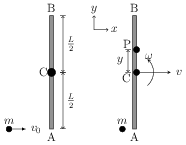# Collision of Point Masses with Rigid Bodies

## Problems from IIT JEE

Problem (IIT JEE 2000): A rod AB of mass $M$ and length $L$ is lying on a horizontal frictionless surface. A particle of mass $m$ travelling along the surface hits the end A of the rod with velocity $v_0$ in the direction perpendicular to AB. The collision is elastic. After the collision the particle comes to rest.

1. Find the ratio $m/M$.
2. A point P on the rod is at rest immediately after collision. Find the distance AP.
3. Find the linear speed of the point P a time $\frac{\pi L}{3v_0}$ after the collision.

Solution:Let C be centre of mass of the rod of mass $M$ and length $L$. Consider the rod and the particle together as a system. Let $v$ be velocity of C and $\omega$ be angular velocity of the rod just after collision. The linear momentum of the system just before and just after the collision is, \begin{align} p_i=mv_0,&&p_f=Mv.\nonumber \end{align} There is no external force on the system in $x$ direction. Hence, linear momentum in $x$~direction is conserved i.e., $p_i=p_f$, which gives, \begin{align} \label{hpb:eqn:1} Mv=mv_0. \end{align} The angular momentum of the system about C just before and just after the collision is, \begin{align} L_i=mv_0 L/2,&&L_f=\omega I_c=M\omega L^2/12.\nonumber \end{align} There is no external torque on the system about C. Hence, angular momentum of the system about C is conserved i.e., $L_i=L_f$, which gives, \begin{align} \label{hpb:eqn:2} mv_0=M\omega L/6. \end{align} The kinetic energy before and after the collision is, \begin{align} &K_i=\tfrac{1}{2}mv_0^2,&K_f=\tfrac{1}{2}Mv^2+\tfrac{1}{2}I_c\omega^2.\nonumber \end{align} Since kinetic energy is conserved in elastic collision, $K_i=K_f$, i.e., \begin{align} \label{hpb:eqn:3} mv_0^2=Mv^2+(ML^2/12)\omega^2. \end{align} Solve above equations to get ${m}/{M}={1}/{4}$, $v={v_0}/{4}$, and $\omega={3v_0}/{(2L)}$.

The velocity of a point P with position vector $\vec{r}_\text{PC}$ from C is given by $\vec{v}_P=\vec{v}_C+\vec{\omega}\times\vec{r}_\text{PC}$. Just after the collision $\vec{r}_\text{PC}=y\,\hat\jmath$. Thus, P is at rest if, \begin{align} \vec{v}_P=\frac{v_0}{4}\;\hat\imath+\left(\frac{3v_0}{2L}\,\hat{k}\right) \times \left(y\,\hat\jmath\right)=\frac{v_0}{4}-\frac{3yv_0}{2L}=0, \end{align} which gives $y={L}/{6}$. The distance $\mathrm{AP}=\mathrm{AC}+\mathrm{CP}={L}/{2}+{L}/{6}={2L}/{3}$. After the collision, C keeps moving with $\vec{v}_C={v_0}/{4}\,\hat\imath$ and angular velocity of the rod remains $\vec{\omega}={3v_0}/{(2L)}\,\hat{k}$. The angular displacement of the rod in time $t={\pi L}/{(3v_0)}$ is $\omega t={\pi}/{2}$ and hence after time $t$ position vector of P w.r.t. C is $\vec{r}_\text{PC}=-{L}/{6}\,\hat\imath+0\,\hat\jmath$. The velocity of P is, \begin{align} &\vec{v}_P=\vec{v}_C+\vec{\omega}\times\vec{r}_\text{PC}=\frac{v_0}{4}\hat\imath+\left(\frac{3v_0}{2L} \,\hat{k}\right)\times \left(-\frac{L}{6}\,\hat\imath\right)=\frac{v_0}{4}\,\hat\imath-\frac{v_0}{4}\,\hat\jmath,\nonumber \end{align} and its magnitude is $|\vec{v}_{P}|=\frac{v_0}{2\sqrt{2}}$.